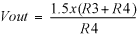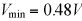# Pushbuttons Control Regulated Switching Converter

### Abstract

Pushbutton control of a digital potentiometer enables this circuit to control the regulated output of a switching converter.

Digitally controlled potentiometers (digipots) are useful for generating analog control voltages, but they usually operate under the direction of a microcontroller. Pushbutton control is simpler, but the digipot's inputs have no switch-debounce capability, and its up/down control is not particularly suitable for pushbuttons. Nor are such kinds of applications (pushbutton control of digipots) supported by application notes. Figure 1 illustrates the issues involved in wiring a digipot to a boost converter.The digipot shown (IC1) has an end-to-end resistance of 100kΩ. To increment the position of the wiper (W), pull the U/D pin high by depressing the U/D pushbutton (S2), and then pulse the INC input by pulsing pushbutton S1. Similarly, you decrement the wiper position by releasing S2 and pulsing S1.

A time-delay network (R1, R2, and C1) masks the switch bounce on S1 that would otherwise toggle the wiper position between VDD and 0V. With S1 depressed, the time required for capacitor C1 to charge via R2 causes the INC pin to ramp slowly to 0V, thereby removing the effect of any bounce that occurs in S1. This time constant on the signal applied to INC requires that you depress S1 for several milliseconds before it has any effect.

IC1 comes in three versions—L, M, and N—corresponding to the end-to-end resistances 50Ω, 100kΩ, and 200kΩ. This circuit uses the M version, but the choice makes little difference. The switching converter (IC2) was wired in the standard boost-converter configuration with an output voltage of 12V. Use the following formula to set the output voltage of IC2 without the digipot connected:Connecting the wiper of IC1 via a 10k resistor to the FB node of IC2 sets that feedback according to the voltage on IC1's wiper. The equation for setting the output voltage precisely is a complex one that includes the resistances of the feedback resistors and of the digipot, but it can be simplified by calculating voltages at the extremes of the wiper setting. Thus, if R' equals the resistance of R4 and R5 in parallel, the maximum IC2 output voltage is set byThe minimum output voltage can be calculated by summing voltages into the feedback node, with the MAX5160 wiper set to 5V:which equates toNote that the output voltage on a boost converter cannot be adjusted below its input voltage. (Otherwise, you might spend an hour wondering why your adjustment voltage does not go down to the calculated 0.48V.) Setting R5 = 10k gives the following empirical values for Vmin and Vmax:

Vmin = 4.93V
Vmax = 16.84V